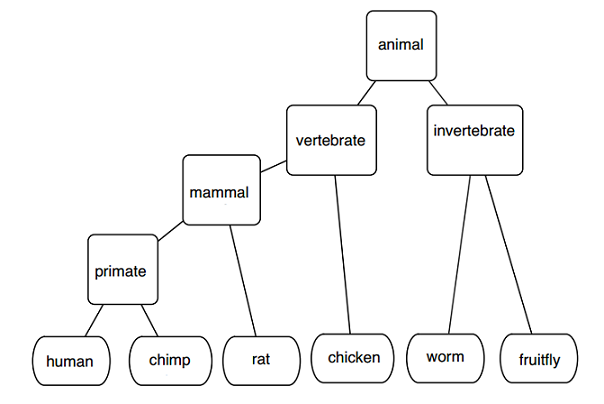Computer Science

# Trees - BasicGiven a tree that describes the evolutionary relationship of a set of organisms, return the two organisms that are the most distantly related evolutionarily. I.e. Out of all organism pairs in the tree, the sum of the distances of organisms A and B from their most recent common ancestor is maximum.

If the distance between two nodes is defined as the number of nodes on the path between the two nodes, then what is the distance between the organisms that are most distantly related?

Given a binary search tree and a list of numbers, write a program to insert the list of numbers into the binary search tree in such a way that the list remains a binary search tree.

Which of the following is a postorder traversal of the binary search tree after the numbers: $8, 10, 12, 14, 17, 19, 21$ are inserted into the below binary search tree?

 1 2 3 4 5 6 7  15 / \ 13 18 / / 9 16 \ 11 

Given the following sorted array, which of the following is a postorder traversal of a balanced binary search tree implementation of the array?

[2, 5, 6, 9, 10, 11, 18, 23, 27, 31, 33, 39]

Given an array of the first $n$ Fibonacci numbers, write a program to construct a balanced binary search tree from the array.

Which of the following is a preorder traversal of balanced binary tree of the first $13$ Fibonacci numbers?

Given a (sorted) array of distinct integers, write a program that constructs a balanced binary tree, and counts the number of nodes in that tree. How many nodes does a balanced binary tree formed from the below array have ?

[1, 3, 4, 6, 7, 9, 10, 13, 15, 16, 19, 24, 27, 28, 29, 30, 34, 37, 39, 41, 43, 44, 46, 48, 49, 51, 52, 54, 55, 59, 69, 70, 72, 77, 81, 84, 85, 86, 88, 89, 90, 93, 95, 98, 99, 102, 104, 106, 108 , 109 , 111, 120, 134, 150, 151, 153, 164, 176, 179, 180, 185, 187, 188, 190, 195, 200, 205, 210, 220, 240, 260, 290, 310, 322, 333, 344, 355, 366, 388, 399]

×